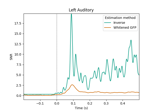# mne.viz.plot_snr_estimate¶

mne.viz.plot_snr_estimate(evoked, inv, show=True, axes=None, verbose=None)[source]

Plot a data SNR estimate.

Parameters
evokedinstance of Evoked

The evoked instance. This should probably be baseline-corrected.

invinstance of InverseOperator

The minimum-norm inverse operator.

showbool

Show figure if True.

axesinstance of Axes | None

The axes to plot into.

New in version 0.21.0.

verbose

If not None, override default verbose level (see mne.verbose() and Logging documentation for more). If used, it should be passed as a keyword-argument only.

Returns
figinstance of matplotlib.figure.Figure

The figure object containing the plot.

Notes

The bluish green line is the SNR determined by the GFP of the whitened evoked data. The orange line is the SNR estimated based on the mismatch between the data and the data re-estimated from the regularized inverse.

New in version 0.9.0.

## Examples using mne.viz.plot_snr_estimate¶